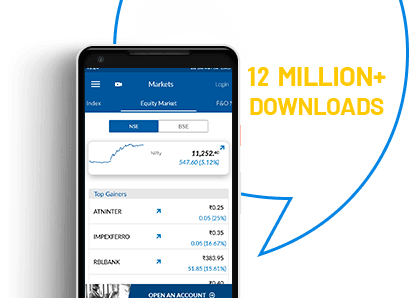#FUTURE VALUE CALCULATOR#### Future Value Calculator

The value of an asset is not constant. It keeps changing over time for various reasons like inflation and the returns earned on it. While inflation steadily erodes the capital, the returns that you earn from it add value. Usually, returns on the capital are higher than inflation; so your asset will grow over time. A future value calculator helps compute such growth.

It is, therefore, important for individuals and businesses to have an understanding of the future value of their assets. The most feasible way of doing it is by using a future value calculator. India has many websites that come with such calculators; they are convenient and user-friendly.

FUTURE VALUE IS

Enjoy FREE Equity Delivery for Lifetime

Why is it essential to calculate future value?
It allows individuals to have an idea of what their savings would be worth a few years from now. A future value calculator online helps them figure out if the value of their savings would be adequate to meet their needs a few years from now. If inadequate, they can take corrective measures by saving more or investing more in higher-yielding assets.
A future value calculator India calculates the future of money and is often used in certificates of deposit, bonds or interest-bearing accounts. It is indispensable to the industry of financial services. Providers of financial products such as home loans, auto loans and credit cards need to know the future value of assets. A future value calculator makes it easy for them to foresee the revenues they could make.

How to calculate future value?
If you are that inclined, you can do the calculations manually without the help of an online calculator. The most basic step would be to calculate simple interest. You don’t need an FV calculator for that.

Future value = P + (P*r*t)
P = Initial value
R= Rate of interest
T= Number of years

A more complex way would be to calculate the value of the compounded asset. In this case, the formula would be:

Future value = P * (1+r)t
P = Initial value
R = Rate of interest
T = Duration for which you want to calculate future value (in years)

What should you do in case of an annuity, where payments are made at regular intervals? If you invest Rs 10,000 in a fixed deposit and keep adding Rs 1,000 to it each year, you may want to find out the value of your investment ten years from now. In that case, you can use a future value of annuity calculator. It has an additional field, where you have to enter the annual incremental sum that you want to make.

FV chart preparation
Your use of an online future value calculator can rightly inspire you to the FV chart preparation tool. The tool helps you to decide on a wise investment plan by examining the time value of your money. It will lead investors to a matrix of FVs for varying interest rates and tenures for a specific sum of money. The chart gives you an overview.

Limitations of the future value calculator
A future value calculator has its limitations. The future is unpredictable. The calculator assumes that rates of return remain constant over time. We all know that this is not entirely true in the real world. Nevertheless, such calculators can give us some idea, and we can use that to make plans in the present.
Want to know the worth of your assets ten years from now? Let a future value calculator assess on your behalf!

### How do you calculate the future value of monthly investments?

Future value of an investment depends on future return, inflation, and tax rates. An investment is worth pursuing if the return offered exceeds future inflation. Use a future value calculator to calculate the FV of your investment. These calculators are lifesavers and calculate the future value with speed and accuracy.

### How do you calculate the future value on a calculator?

A future value calculator works on user input. You would have to feed it with simple inputs like initial investment amount, return on investment (%), rate of inflation (%), duration of investment (in years). There are many such FV calculators available on the internet. These calculators are user-friendly and used for a variety of purposes.

### How do you calculate the future value of a series of deposits?

You can use a future value calculator with compounding calculation feature. There is a wide variety of future value calculators available on Indian websites. These calculators are simple to use and function on user input.

### Why do you need to calculate future value?

Future value refers to the value of a current asset on a future date, calculated on an assumed rate of return – also called the time value of money. It is useful during financial planning to help you gauge how much you are going to receive at a future date if you invest Rs X amount today. Accordingly, you can adjust your current financial goals.
Future value calculation is widely used by investors and businesses alike to determine ROI.

### What two methods are used to calculate future values?

There are simple interest future value and compound interest FV and the calculation of both are slightly different from each other.
FV for simple interest uses the following formula.
FV = PV (1 + r)n
Where,
PV stands for the present value of money
r is the rate of interest
n equals the duration of the investment

The formula for calculating the future value of an investment at a compounding rate is a little different. A classic example of compounding future value is an annuity, where a certain amount is invested for over a period. Let’s take a look at it.

FVOA = A ×(1 + r)n – 1
R
Using a future value of the annuity calculator is an easy alternative to calculate the complex formula.

Enjoy Zero Brokerage on Stock Investments